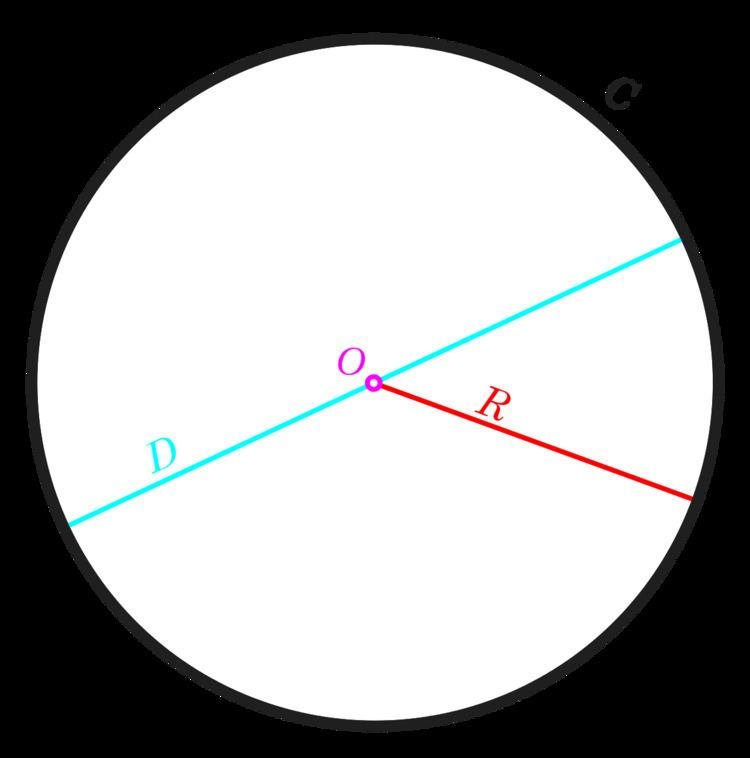# Centre (geometry)

Updated on
Covid-19In geometry, a centre (or center) (from Greek κέντρον) of an object is a point in some sense in the middle of the object. According to the specific definition of centre taken into consideration, an object might have no centre. If geometry is regarded as the study of isometry groups then a centre is a fixed point of all the isometries which move the object onto itself.

## Circles, spheres, and segments

The centre of a circle is the point equidistant from the points on the edge. Similarly the centre of a sphere is the point equidistant from the points on the surface, and the centre of a line segment is the midpoint of the two ends.

## Symmetric objects

For objects with several symmetries, the centre of symmetry is the point left unchanged by the symmetric actions. So the centre of a square, rectangle, rhombus or parallelogram is where the diagonals intersect, this being (amongst other properties) the fixed point of rotational symmetries. Similarly the centre of an ellipse or a hyperbola is where the axes intersect.

## Triangles

Several special points of a triangle are often described as triangle centres:

• the circumcentre, which is the centre of the circle that passes through all three vertices;
• the centroid or centre of mass, the point on which the triangle would balance if it had uniform density;
• the incentre, the centre of the circle that is internally tangent to all three sides of the triangle;
• the orthocentre, the intersection of the triangle's three altitudes; and
• the nine-point centre, the centre of the circle that passes through nine key points of the triangle.
• For an equilateral triangle, these are the same point, which lies at the intersection of the three axes of symmetry of the triangle, one third of the distance from its base to its apex.

A strict definition of a triangle centre is a point whose trilinear coordinates are f(a,b,c) : f(b,c,a) : f(c,a,b) where f is a function of the lengths of the three sides of the triangle, a, b, c such that:

1. f is homogeneous in a, b, c; i.e., f(ta,tb,tc)=thf(a,b,c) for some real power h; thus the position of a centre is independent of scale.
2. f is symmetric in its last two arguments; i.e., f(a,b,c)= f(a,c,b); thus position of a centre in a mirror-image triangle is the mirror-image of its position in the original triangle.

This strict definition excludes pairs of bicentric points such as the Brocard points (which are interchanged by a mirror-image reflection). The Encyclopedia of Triangle Centers lists over 9,000 different triangle centres.

## Tangential polygons and cyclic polygons

A tangential polygon has each of its sides tangent to a particular circle, called the incircle or inscribed circle. The centre of the incircle, called the incentre, can be considered a centre of the polygon.

A cyclic polygon has each of its vertices on a particular circle, called the circumcircle or circumscribed circle. The centre of the circumcircle, called the circumcentre, can be considered a centre of the polygon.

If a polygon is both tangential and cyclic, it is called bicentric. (All triangles are bicentric, for example.) The incentre and circumcentre of a bicentric polygon are not in general the same point.

## General polygons

The centre of a general polygon can be defined in several different ways. The "vertex centroid" comes from considering the polygon as being empty but having equal masses at its vertices. The "side centroid" comes from considering the sides to have constant mass per unit length. The usual centre, called just the centroid (centre of area) comes from considering the surface of the polygon as having constant density. These three points are in general not all the same point.

## References

Similar Topics
A Letter from Bataan
Remko Bicentini
Boris Martinec
Topics